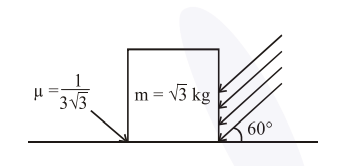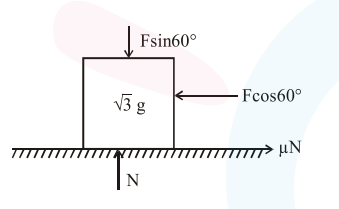# As shown in the figure, a block of mass

Question:

As shown in the figure, a block of mass $\sqrt{3} \mathrm{~kg}$ is kept on a horizontal rough surface of

coefficient of friction $\frac{1}{3 \sqrt{3}}$. The critical force

to be applied on the vertical surface as shown at an angle $60^{\circ}$ with horizontal such that it does not move, will be $3 \mathrm{x}$. The value of $\mathrm{x}$ will be

$\left[\mathrm{g}=10 \mathrm{~m} / \mathrm{s}^{2} ; \sin 60^{\circ}=\frac{\sqrt{3}}{2} ; \cos 60^{\circ}=\frac{1}{2}\right]$Solution:$\mathrm{F} \cos 60^{\circ}=\mu \mathrm{N}$ or $\frac{\mathrm{F}}{2}=\frac{1}{3 \sqrt{3}} \mathrm{~N} \ldots$ (1)

$\& \mathrm{~N}=\sin 60^{\circ}+\sqrt{3} g \quad \ldots$(2)

$\frac{\mathrm{F}}{2}=\frac{1}{3 \sqrt{3}}\left(\frac{\mathrm{F} \sqrt{3}}{2}+\sqrt{3} \mathrm{~g}\right)$

$\Rightarrow \mathrm{F}=\mathrm{g}=10$ Newton $=3 \mathrm{x}$

So $x=\frac{10}{3}=3.33$